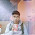## Chemistry

### Quantum Numbers - Simplified Notes

Quantum numbers are like the address of an electron. It tells where an electron is located around the nucleus of the atom. Just just we have home addresses, even electrons have an address of their location. Some parts of our address are not so specific of our location whereas other parts are. So, quantum numbers are like lines of your home address.There are 4 quantum numbers.

## Principal Quantum Number (n)

This is like the city name. It is not very specific about the location of the electron.
This number describes the energy level/orbit/shell in which the electron is located. (energy level is the same thing as orbit or shell)

Neil Bohr said that the atom has various energy levels around the nucleus. The principal quantum number tells us in which energy level the electron is located.

There can be many energy levels around the nucleus but normally we only deal with the first 4 or 5.

∴ n=1, 2, 3, 4.....
There are names given to the shells.

n=0 → shell
n=1 → shell
n=2 → shell
n=3 → n shell

## Azimuthal or Subsidary Quantum Number (l)

This quantum number is like the name of the street in our address. It is more specific as compared to the principal quantum number.

This Quantum number describes the subshells which are present within a shell. Moreover, it also describes the shape of the orbitals about which we will talk about later.

There are a specific number of subshells present within each shell. Hence the number of subshells in a shell is related to shell number which is described by the principal quantum number as follows:

l= 0n-1 (read as l is equal to all values from zero to n minus one)
So for a given quantum number n, l can be any value from 0n-1
Example: Principal quantum number 3
∴ l=0n-1
∴ l=03-1
∴ l=02
∴ l can take up value 0, 1, 2

Example: Principal quantum number 4

∴ l=0n-1
∴ l=04-1
∴ l=03
∴ l can take up value 0, 1, 2, 3

Here 0 is counted as a subshell and hence it means something.
l=0 s-subshell                       l=2 d-subshell
l=1 → p-subshell                       l=3 f-subshell

∴The 3rd shell has 3 subshells- s, p, d
and the 4th shell has 4 subshells s, p, d, f

We also observer that if we move up by one shell we add subshell just like in the above examples.

## Magnetic Quantum Number (m)

First come the shells. Within the shells are located the subshells and withing the subshells are located orbitals! The magnetic quantum number represented by 'm' tells us which orbital the electron is located in. This like the house number of the electron.
This quantum number tells us the orientation.

NOTE: EACH ORBITAL CAN ACCOMMODATE A MAXIMUM OF 2 ELECTRONS.

Since orbitals are within subshells, there is a mathematical representation between the subshells and orbitals. This is given by:
m= -l→ +l (m equals all values from -ive l to +ive l)
This includes 0.
Example: For p subshell
since we are given p subshell, l=1
∴ m= -1 → +l
∴ m= -1, 0, 1

∴ there are 3 orbitals within subshell p.

∴ since each orbital can accommodate a maximum of 2 electrons and p subshell has 3 orbitals, we conclude that p subshell can accommodate a maximum of 6 electrons.

Example: How many electrons can there in s-subshell?
For s-subshell, l=0.
∴ m= -0 → +0

∴ m= 0

There is only one orbital within s-subshell.
(0 doesn't mean that there are zero orbitals. 0 is considered as 1 orbital!)

∴ since each orbital can accommodate a maximum of 2 electrons and s-subshell has 1 orbital, we conclude that s-subshell can accommodate a maximum of 2 electrons.

If you observe carefully you will notice a relation between the shell and the number of orbitals present in it. This is:

number of orbitals present in a shell=n2
where n is the shell number, i,e, k, l , m, n, etc.

Here another important relation:

The maximum number of electrons that can occupy a specific energy level is 2n2

where n is the shell number. This is just a continuation of the previous relation if you think about how that 2 appeared there.

## Spin Quantum Number (s)

This is the simplest kind of quantum number. It basically tells us in which direction the electron is spinning. There can be only 2 values of the spin quantum number, i,e, -1/2 and +1/2. And the awesome part is that there is no mathematical relation to find the spin quantum number. We cant exactly determine the direction of its spin.

-1/2 means that the electron is spinning downwards.
+1/2 means that the electron is spinning upwards.

s= 1/2 or +1/2.

In subjective tests, for this quantum number its better to mention both 1/2 and +1/2 because we cannot calculate the direction of spin. In objective tests if you get the other 3 quantum numbers it really doesn't matter weather the option says 1/2 or +1/2.

Now we draw to the end of this chapter. But we are missing one thing. How do we compile the address of the electron and write it in an easily understandable way? Here's how:

Example: If the electron is present in n=3 and l=2.

we know that n=3 and l=2
we write this as 3d
were 3 is the shell number (n) and d is the subshell number (l).

We can calculate the number of orbitals present in l subshell by using the relation m= -l→ +l. In this case we get 5 possibilities of m, i,e, -2, -1, 0, +1, +2.
Since the electron can be in any of these orbitals we don't represent them.
We also know that the maximum electrons present in the d-subshell is 6. So a completely filled 3d is represented by 3d6.

1.2.3.4.